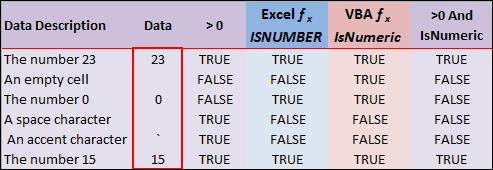Excel ISNUMBER or IsNumeric Function

I don’t like it when Excel worksheet functions are different from their VBA counterparts. Makes for some aggravation.

For example, I have a user generated data range that’s supposed to be made up of either numbers or empty cells. However, Excel users sometimes bump their keyboards inadvertently (when you hit the space bar) and things like a space character can get entered into an otherwise empty cell, and go undetected to the naked eye.

VBA Programming

As part of a VBA macro I looped through each cell in this data range and performed an operation for any cell value greater than zero. This was a good plan for a data range having only number values or empty cells. (Well, not really as you’ll see below.)

However, I not only came across a space character in the user data, but also an accent character (`). As you can see in third column of the the chart pictured below, (>0) greater than zero is TRUE for the space character and the accent mark.What I really wanted was the Excel ISNUMBER fucntion that only shows TRUE when, well, there’s a number. (Fourth column)

So I tried the VBA Excel IsNumeric function in my macro, thinking it to be the same thing, and found that it didn’t work as I had imagined. For some reason the IsNumeric function shows TRUE for and empty cell as you can see in fifth column of the chart.

I wrote a function called IsNum() to simulate the VBA function IsNumeric in the worksheet.

Function IsNum(data As Variant)
If IsNumeric(data) Then
IsNum = True
Else
IsNum = False
End If
End Function

The difference seems to be that the Worksheet Function ISNUMBER takes a Value for an argument, and the VBA function IsNumeric takes an Expression for its argument.

My solution is to use both greater than zero (>0) AND IsNumeric for my logic gate to perform further operations. (Last column in the chart) So in looping through the data range above, operations would only be performed on the numbers (23 and 15).

Lessons Learned About Excel ISNUMBER or IsNumeric Function

1. Now I know that an accent character (`) is greater than zero.
2. The VBA function IsNumeric is different than the Excel worksheet function ISNUMBER.
3. Testing doesn’t always bring out all the ways a user can change the data. Only time and more users can do that.
4. One logical operation in a very large VBA macro (9 subroutines w/ 2 functions) can bring down the whole show.7 thoughts on “Excel ISNUMBER or IsNumeric Function”

1.Marco

Hi,
You will need more then adding ” >0 And ” in the code
If the data in a cell has a value of : ‘1
Excel function IsNumber = False
VBA function IsNumeric = True
>0 and IsNumeric = True
If the data cell is formatted as ‘text’ and contains value 1:
(in this case the value will not be taken into consideration with the excel “SUM” function)
Excel function IsNumber = False
VBA function IsNumeric = True
>0 and IsNumeric = True

BR.Marco

1.Gregory

@Marco, having to work with something like ‘1 is why Excel developers get headaches. When ISNUMBER and IsNumeric can’t agree it makes things even more complicated. Thanks for pointing out the discrepancy.

2.Besh

You can use Len function to test, whether the cell is empty first and then used IsNumeric to test for numeric values. This can manage the problem with empty cells.

Dim L as integer
L = Len(cells(row, culumn)) = 0

Select case L
Case is = 0
‘set output as false
case is >0
‘use IsNumeric function
end select

1.Gregory

Actually, that works pretty darn good. Thanks.

Function IsNum2(data As Variant)
Dim L As Integer
L = Len(data)
Select Case L
Case Is = 0
IsNum2 = False
Case Is > 0
If IsNumeric(data) Then
IsNum2 = True
Else
IsNum2 = False
End If
End Select
End Function

3.Mike

isnumber() is available if you use the following line in your code

Application.worksheetfunction.isnumber()

1.Gregory

Thanks for reminding me.

For some reason, which I don’t clearly state in my blog post, I remember not using that line of code, but didn’t want to confuse the issue. I think it had to do with the range of data I was testing was dumped into an Array and the application.worksheetfunction.isnumber function wouldn’t work, so I had to think up something else or re-write a good bit of code.

4.Eldar Agalarov

Use this function:

Public Function IsNumber(ByRef expression As Variant) As Boolean
IsNumber = Not IsEmpty(expression) And IsNumeric(expression)
End Function

And have fun!